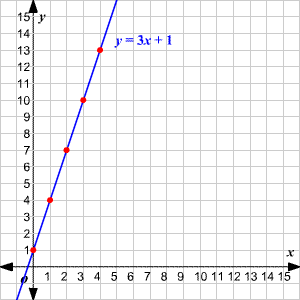# Input-Output Tables

An input-output table is often used to generate a set of ordered pairs for a function , when the rule is known.

Example 1:

 $x$ $0$ $1$ $2$ $3$ $4$ $y=3x+1$ $1$ $4$ $7$ $10$ $13$

Here, the function rule is $y=3x+1$ .

The first input is $0$ . Since $3\left(0\right)+1=1$ , the first output is $1$ .

The second input is $1$ . Since $3\left(1\right)+1=4$ , the second output is $4$ .

The $5$ ordered pairs in this table can be plotted and used to graph the equation, as shown.Alternatively, you may be presented with an input-output table and asked to guess the rule for the function.

Example 2:

Guess the function rule.

 $x$ $10$ $16$ $26$ $100$ $200$ $y$ $4$ $7$ $12$ $49$ $99$

With a little thought you can guess that:

$y=\frac{1}{2}x-1$

Note that for this kind of problem, you can never say for sure what the function rule is, since for any finite set of ordered pairs, there are many different functions that go through them. But usually there is a fairly obvious one that you can guess.